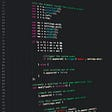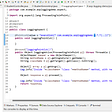# Calculus using Functional Python

The aim this piece is to demonstrate some concepts of functional programming in Python, let’s dive-in!

Part Derivative:

The following function calculates the slope on a non-linear line.

Now let rewrite the code in Python.

`derivative = lambda f, x: ((f(x + 1e-3) - f(x)) / 1e-3)`

This function is equivalent to the prior:

`def derivative (f, x):    return ((f(x + 1e-3) - f(x)) / 1e-3)`

Functions in Python are first-class functions, which means that you can treat them as variables.

Note that the value 1e-3 (0.001) is the limit when h approaches zero (h → 0).

Now we use a partial function:

`derivative_of_sin = partial(derivative, math.sin)`

Partial functions allow us to pass fewer arguments to multiple arguments function.

In this example, we create a partial function from the function derivative, and its first argument (the sine function), leaving x for call time.

`x = 5derivative_of_sin(x) # --> 0.28math.cos(x)          # --> 0.28`

Correct! since the derivative of sin is cos.

Let’s try x²

`derivative_of_x2 = partial(derivative, lambda a: a * a)`

As you can see, we passed a lambda calculus expression because our function derivative expects a function.

`derivative_of_x2(x) # --> 10`

Ten is the correct answer since the derivative of is 2 * x.

Part Integral:

We are going to use algebra to tackle the integral function.

This function has four important arguments; the range from a to b, the steps, and the input function.

In calculus, we find the integral of (x³ / 3) to find the solution.
With algebra, we stack multiple rectangles together to find the solution.

Using algebra:

`| steps     | equation                     |   result ||-----------+------------------------------+----------|| 100       | (1e-1) * (10^2 + ... + 20^2) | 2318.349 || 1_000     | (1e-2) * (10^2 + ... + 20^2) | 2331.833 || 10_000    | (1e-3) * (10^2 + ... + 20^2) | 2333.183 || 100_000   | (1e-4) * (10^2 + ... + 20^2) | 2333.318 || 1_000_000 | (1e-5) * (10^2 + ... + 20^2) | 2333.331 ||           |                              |          |*steps count = (20 - 10) / 1e-5 = 1_000_000`

Using calculus:

`(1 / 3) * (20**3) - (1 / 3) * (10**3) # --> 2333.33`

The algebra method is approaching the correct solution, albeit it requires a lot of calculation than you transistors!

Let’s define the function:

`def integral (f, a, b):    delta = 1e-6    auc = 0    for i in np.arange(a, b, delta):        auc = auc + f(i)    return auc * delta`

Note that AUC stands for the area under the curve.

Integral is a high-order function, which means that it can take a function as an argument or return a function.

Now, let’s use partial again:

`integral_of_x2 = partial(integral, lambda x: x**2)`

if we pass our partial function with the limits a and b, we get:

`integral_of_x2(10, 20)    # ==> 2333.331`

Hooray!

Part Integral++:

Another approach is using closures:

`def integral (f, s):    delta = s    def g (a, b):        auc = 0        for i in np.arange(a, b, delta):            nonlocal auc            auc += f(i)        return auc * delta    return g`

The integral function will return the function g.

The function g holds the value of delta way after the execution of the outer function; this is closure.

Next, we pass a complex function and the number of steps delta.

`integral_of_complex = integral(lambda x: (5*x**2) - (8*x) + 5, 1e-5)`

Passing the limits:

`integral_of_complex2(10, 20) # --> 10515.66`

That’s correct!

Final thoughts:

Functional programming has many elegant concepts, some concepts covered:

• first-class functions
• partial functions
• lambda calculus
• high-order functions
• closures

You can find more examples on the GitHub link with additional functional techniques.

https://github.com/booletic/medium/blob/34b60709ce0bd0d657e1e2c88a58125a19b6ee91/calculus.ipynb

--

--

--

## More from Mansoor Aldosari

https://github.com/booletic

Love podcasts or audiobooks? Learn on the go with our new app.

## Network interoperability provides the core foundation for interoperability between systems.## Top 10 Flutter Benefits In Mobile And IoT Development## HOW NETFLIX IS HANDLING SO MANY MOVIES AND WEBSERIES, THAT YOU BINGE WATCH!## How to Auto Scale within CloudFormation## Python itertools Module Part 2## Spring Boot AOP Example## Mansoor Aldosari

https://github.com/booletic

## Calculus — Curves## The rewrite function in SymPy## Geogebra 4 Engineer## Revisiting Linear Algebra with image filters and python